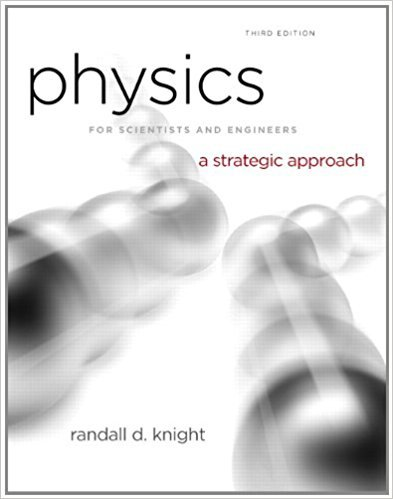×
Get Full Access to Physics For Scientists And Engineers: A Strategic Approach With Modern Physics - 3 Edition - Chapter 2 - Problem 34p
Get Full Access to Physics For Scientists And Engineers: A Strategic Approach With Modern Physics - 3 Edition - Chapter 2 - Problem 34p

×

# A particle’s acceleration is described by the function axISBN: 9780321740908 69

## Solution for problem 34P Chapter 2

Physics for Scientists and Engineers: A Strategic Approach with Modern Physics | 3rd Edition

• Textbook Solutions
• 2901 Step-by-step solutions solved by professors and subject experts
• Get 24/7 help from StudySoup virtual teaching assistantsPhysics for Scientists and Engineers: A Strategic Approach with Modern Physics | 3rd Edition

4 5 1 299 Reviews
13
2
Problem 34P

Problem 34P

A particle’s acceleration is described by the function ax = (10 − 1) m/s2, where t is in s. Its initial conditions are x 0 = 0 m and v 0x = 0 m/s at t = 0 s.

a. At what time is the velocity again zero?

b. What is the particle’s position at that time?

Step-by-Step Solution:

Solution 34P

Step 1 of 2

Part a

We are required to calculate the time when the velocity will be zero.

The acceleration function is given as,m/s2When,Thus,When the velocity is,s

The velocity will be zero when the time is t = 20 s.

Step 2 of 2

##### ISBN: 9780321740908

Unlock Textbook Solution